# CIE A Level Maths: Mechanics复习笔记3.3.1 Resolving Forces & Inclined Planes

### Resolving Forces

If a single force is applied at an angle to the direction of motion it will need to be resolved into components of the force that act perpendicular to each other.

#### Why resolve a force acting at an angle?

• Resolving a force acting at an angle often helps to simplify a problem
• The components of a force parallel and perpendicular to the line of motion allows different types of problems to be solved
• The parallel component of a force acting directly on a particle will be the component that causes an effect on the particle
• The perpendicular component of a force acting directly on a particle will be the component that has no effect on the particle

• Finding the horizontal and vertical components of a force can help solve problems acting in the horizontal plane
• The two components of the force will have the same combined effect as the original force

#### How do you resolve a force acting at an angle?

• Use trigonometry to resolve a force acting at an angle
• Draw a triangle of forces by decomposing the force into its horizontal and vertical components
• The original force will be the hypotenuse of the triangle and the two components will make up the opposite and adjacent sides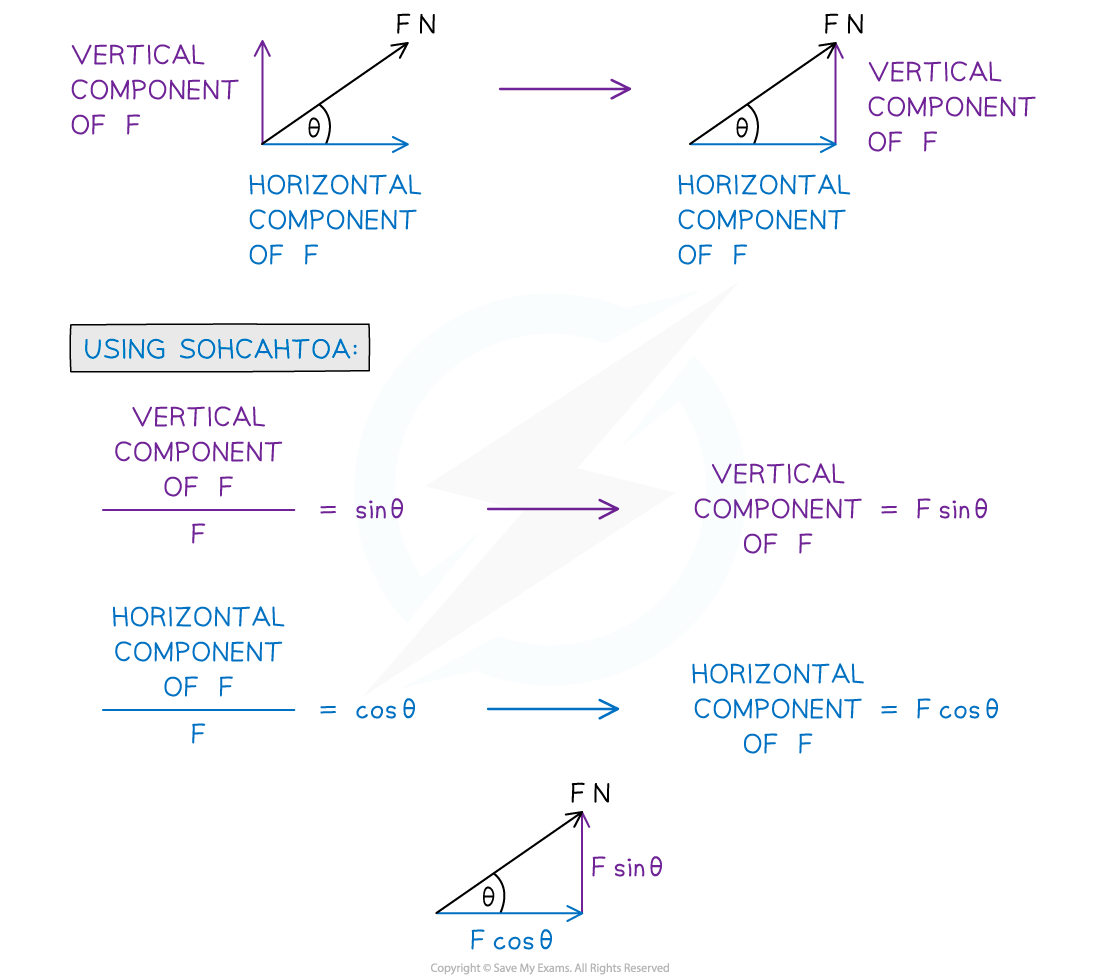#### How do we find a resultant force when two or more forces are acting at angles to each other?

• It is possible to use geometry to find the resultant force without decomposing the forces into their horizontal and vertical components
• The forces can be placed nose to tail to create a vector triangle and then the third side of the triangle can be used to calculate the magnitude and direction of the resultant force
• You will need to use trigonometry to find the missing side or angle in the triangle.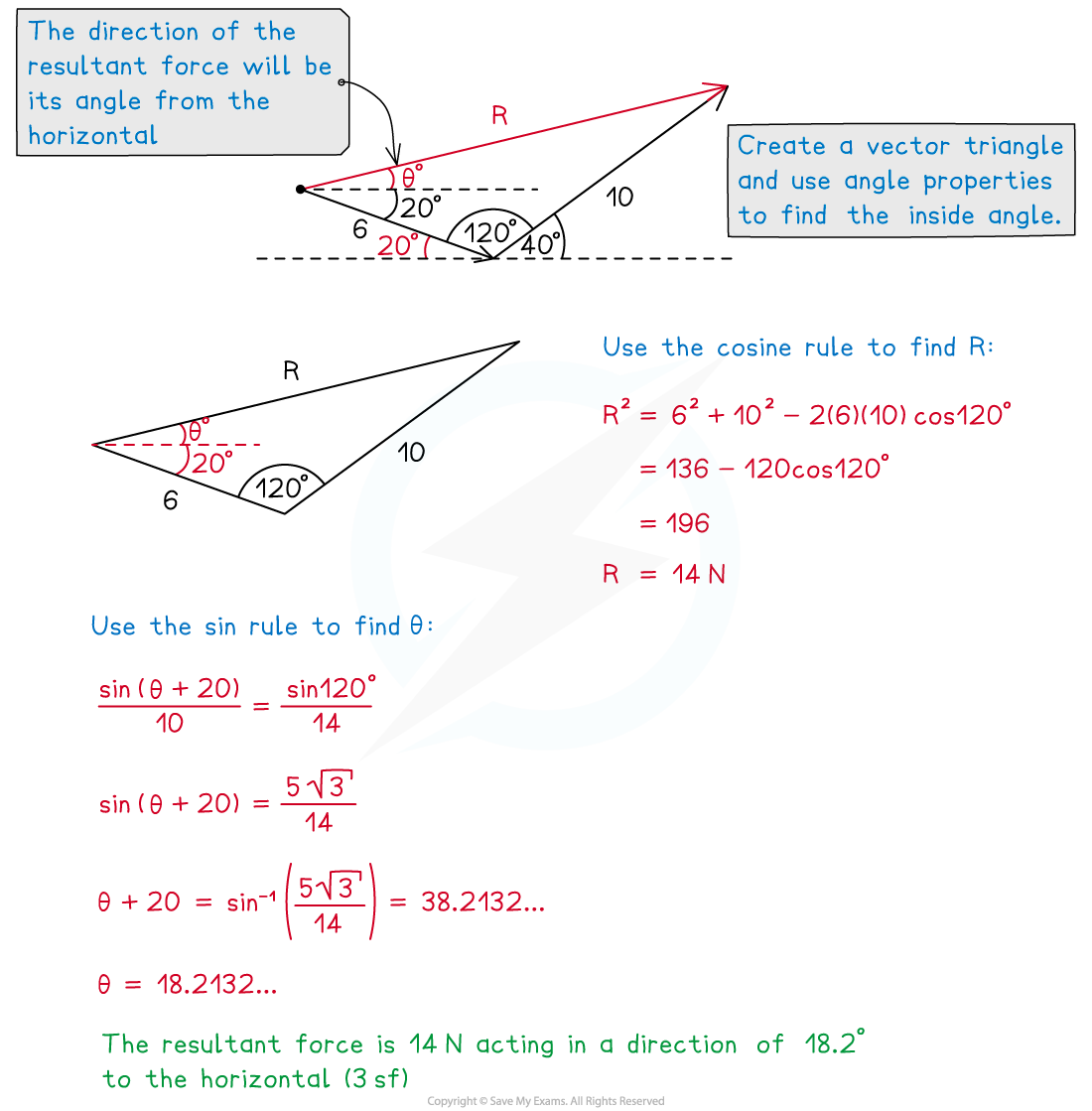#### Worked Example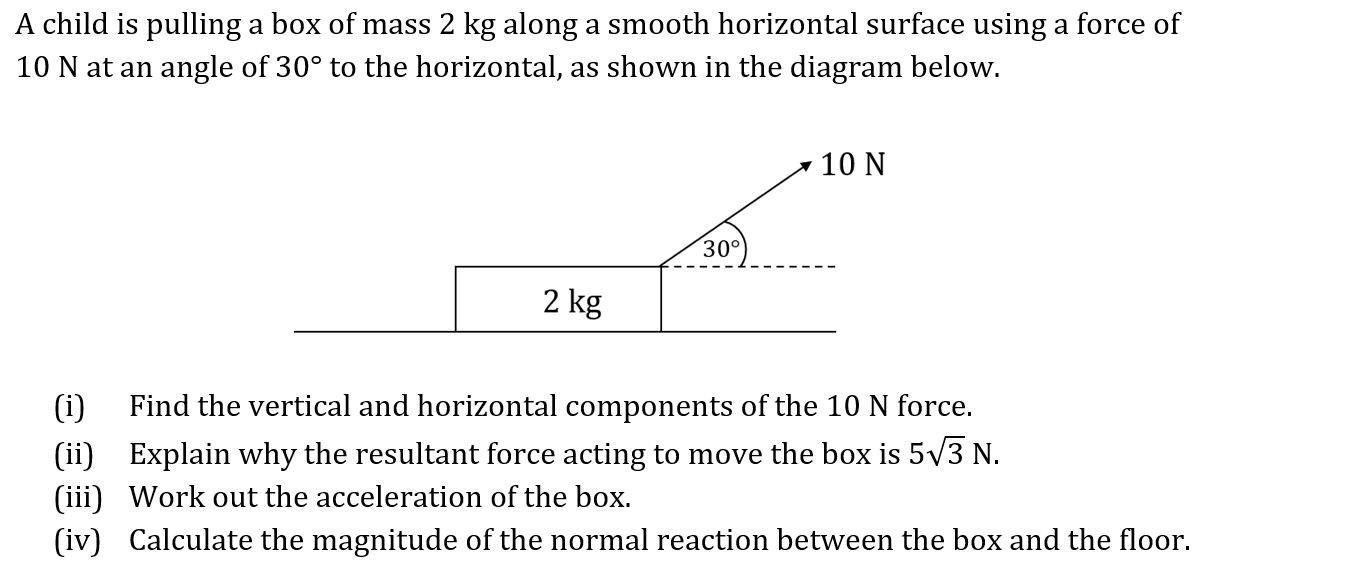1. Find the vertical and horizontal components of the 10 N force.
2.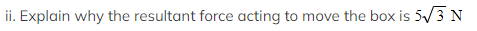Work out the acceleration of the box.
3. Calculate the magnitude of the normal reaction between the box and the floor.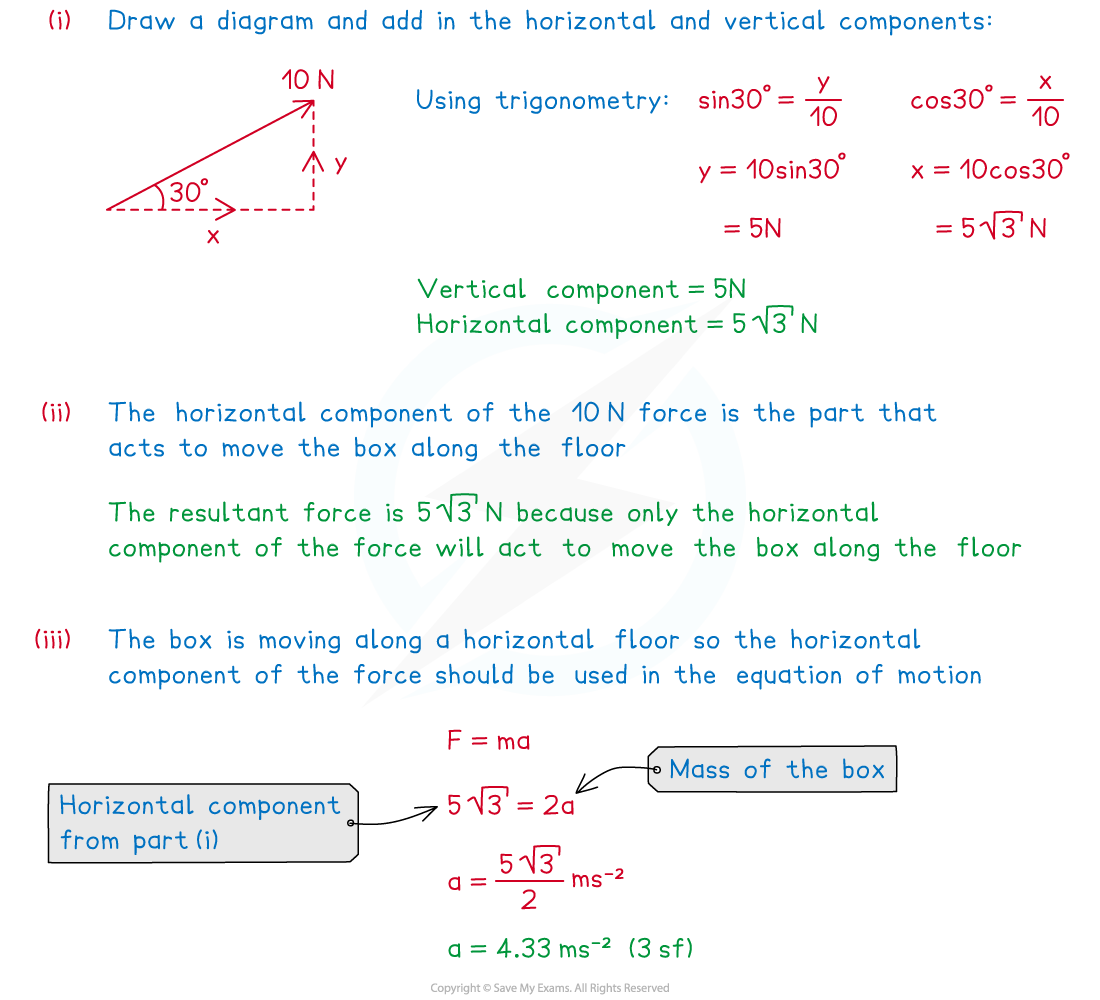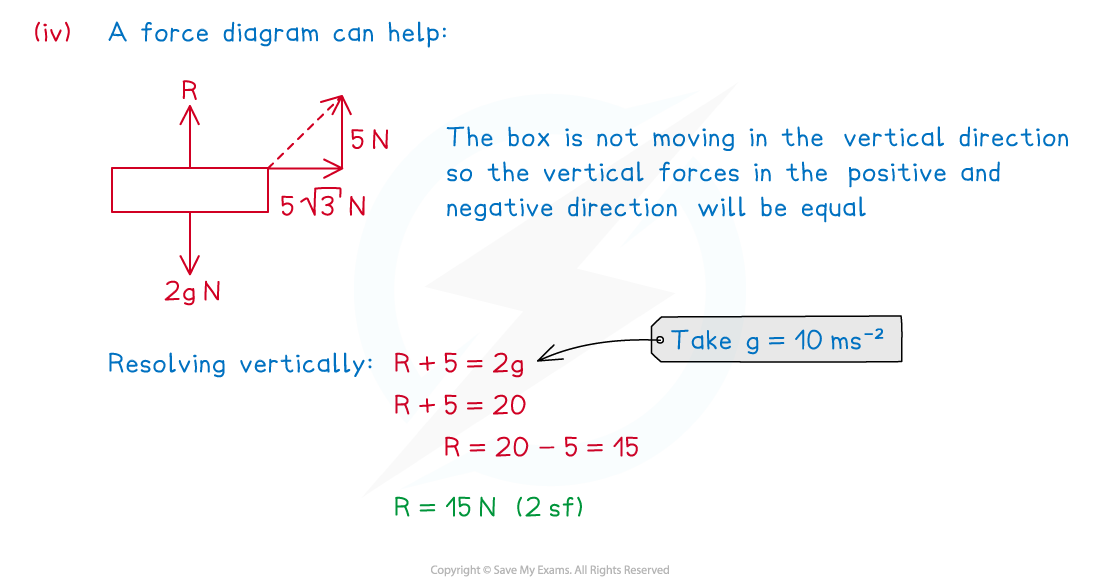#### Exam Tip

• Always draw a diagram and decompose any angled forces into their horizontal and vertical components. Make sure you are confident with basic trigonometry and angle properties.

### Inclined Planes

#### How do you solve a problem involving an inclined plane?

• Problems involving inclined planes will always be acting along the line of greatest slope of the plane
• The line of greatest slope is the most direct route with which it is possible to move from the bottom to the top of the plane
• A force said to be acting in the same vertical plane as the line of greatest slope will be acting in the same plane as this most direct route
• It is usually easier to resolve parallel and perpendicular to the plane
• The normal reaction force always acts perpendicular to the plane and will not need to be resolved
• Weight (mg) always acts vertically downwards and so it is easier to use when resolved into components perpendicular and parallel to the plane
• When an object is moving up or down the inclined plane, it has acceleration only in the parallel direction
• The perpendicular forces will be equal in the positive and negative directions
• Newton’s Second Law (F = ma) can be used in the parallel direction to determine acceleration or missing forces
• On an inclined plane friction will always act in the opposite direction to the direction the object is moving in or on the point of moving in

#### Worked Example

A box of mass 5 kg is being pushed up a smooth ramp inclined at 10° to the horizontal by a force of 12 N.

(i)Find the magnitude of the reaction force acting on the box.
(ii)Find the acceleration of the box up the slope.#### Exam Tip

• Use a triangle of components on your force diagrams to help you work out the components of the force but be careful not to count the components as two separate forces. Make sure you are confident with angle properties and always check you are using the correct angle between the force and the direction you are resolving in.